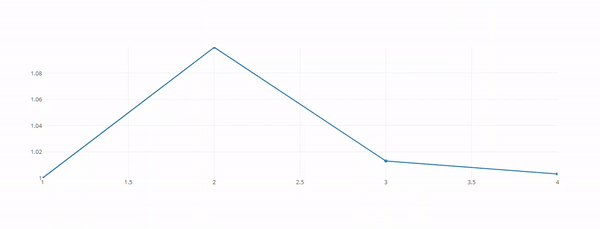# Plot Live Graphs using Python Dash and Plotly

Now, let’s build a dashboard that generates random data, appends it onto a buffer at regular intervals and visualizes the same.

Install Dash module and Plotly modules.

```pip install dash
pip install plotly
```

First, let’s import all the required modules and dependencies. Import Output and Input for callbacks, dash_core_components for graphs and other basic components offered by Dash. Import dash_html_components offers basic HTML components. Also, import dash and plotly. Import the graph_objs from plotly for graph features. Import deque(Doubly Ended Queue) from collections.

```import dash
from dash.dependencies import Output, Input
import dash_core_components as dcc
import dash_html_components as html
import plotly
import random
import plotly.graph_objs as go
from collections import deque```

Let’s initialize two deques for X and Y components of the graph. Append single data to it, this will be our first points on the graph.

```X = deque(maxlen = 20)
X.append(1)

Y = deque(maxlen = 20)
Y.append(1)```

Initialize the dash app.

`app = dash.Dash(__name__)`

Now let’s specify the layout of the dashboard that we want to build. It is important to note that there is no need to write HTML pages for the layout and we can use dash’s HTML components to make simple layouts. Let’s build a simple layout with just a graph component. In the code below, let’s set animate to True, this will easily handle scroll animations for the graph which would look better than an abrupt change in the values after updating. Let’s assign an id to the graph component. We have another component named Interval, which has properties like id which specifies a unique ID for this component. The property interval specifies the time elapsed between two updations of data. n_interval refers to the number of intervals completed from the start of the server.

```app.layout = html.Div(
[
dcc.Graph(id = 'live-graph',
animate = True),
dcc.Interval(
id = 'graph-update',
interval = 1000,
n_intervals = 0
),
]
)```

Now let’s call the callback decorators

```@app.callback(
Output('live-graph', 'figure'),
[ Input('graph-update', 'n_intervals') ]
)```

Now let’s make the update_graph method which takes in n_intervals as parameter. Let’s update X values sequentially, i.e., from 1 to 2 to 3 and so on. Let’s update Y values to random values. Now let’s make a new data variable and assign it to a plotly graph. Plotly graphs, by default, requires you to set X and Y values with a list. Let’s specify the mode to “lines+markers” which essentially means that Plotly is going to plot markers first and then draw lines to it.

```def update_graph_scatter(n):
X.append(X[-1]+1)
Y.append(Y[-1]+Y[-1] * random.uniform(-0.1,0.1))

data = plotly.graph_objs.Scatter(
x=list(X),
y=list(Y),
name='Scatter',
mode= 'lines+markers'
)

return {'data': [data],
'layout' : go.Layout(xaxis=dict(
range=[min(X),max(X)]),yaxis =
dict(range = [min(Y),max(Y)]),
)}```

Finally, run the server.

```if __name__ == '__main__':
app.run_server()```

Complete Code:

## Python3

 `import` `dash ` `from` `dash.dependencies ``import` `Output, ``Input` `import` `dash_core_components as dcc ` `import` `dash_html_components as html ` `import` `plotly ` `import` `random ` `import` `plotly.graph_objs as go ` `from` `collections ``import` `deque ` ` `  `X ``=` `deque(maxlen ``=` `20``) ` `X.append(``1``) ` ` `  `Y ``=` `deque(maxlen ``=` `20``) ` `Y.append(``1``) ` ` `  `app ``=` `dash.Dash(__name__) ` ` `  `app.layout ``=` `html.Div( ` `    ``[ ` `        ``dcc.Graph(``id` `=` `'live-graph'``, animate ``=` `True``), ` `        ``dcc.Interval( ` `            ``id` `=` `'graph-update'``, ` `            ``interval ``=` `1000``, ` `            ``n_intervals ``=` `0` `        ``), ` `    ``] ` `) ` ` `  `@app``.callback( ` `    ``Output(``'live-graph'``, ``'figure'``), ` `    ``[ ``Input``(``'graph-update'``, ``'n_intervals'``) ] ` `) ` ` `  `def` `update_graph_scatter(n): ` `    ``X.append(X[``-``1``]``+``1``) ` `    ``Y.append(Y[``-``1``]``+``Y[``-``1``] ``*` `random.uniform(``-``0.1``,``0.1``)) ` ` `  `    ``data ``=` `plotly.graph_objs.Scatter( ` `            ``x``=``list``(X), ` `            ``y``=``list``(Y), ` `            ``name``=``'Scatter'``, ` `            ``mode``=` `'lines+markers'` `    ``) ` ` `  `    ``return` `{``'data'``: [data], ` `            ``'layout'` `: go.Layout(xaxis``=``dict``(``range``=``[``min``(X),``max``(X)]),yaxis ``=` `dict``(``range` `=` `[``min``(Y),``max``(Y)]),)} ` ` `  `if` `__name__ ``=``=` `'__main__'``: ` `    ``app.run_server()`

Open the browser and run the app on the local host and port 8050

`http://localhost:8050/`

Output:This is how dash can be used for live graph visualizations. In this article, we have used it with random values generated by the computer. However, the same can be done with data pulled from APIs or a database.

My Personal Notes arrow_drop_upCheck out this Author's contributed articles.

If you like GeeksforGeeks and would like to contribute, you can also write an article using contribute.geeksforgeeks.org or mail your article to contribute@geeksforgeeks.org. See your article appearing on the GeeksforGeeks main page and help other Geeks.

Please Improve this article if you find anything incorrect by clicking on the "Improve Article" button below.

Article Tags :

2

Please write to us at contribute@geeksforgeeks.org to report any issue with the above content.